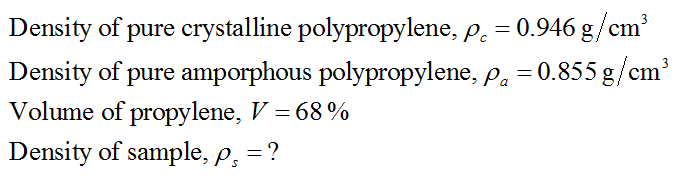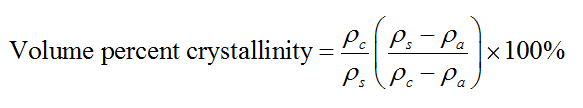# You are given a sample of polypropylene and wish to find out the volume % crystallinity. You know that the density of 100% crystalline polypropylene (ρc) is 0.946 g/cm3, and the density of 100% amorphous polypropylene (ρa) is 0.855 g/cm3. Through your careful measurements, you determine the density of the sample (ρs) is 0.93 g/cm3. Use the following equation to determine the volume % crystallinity (ν) of your sample. Enter your answer with 1 decimal place.Answer is 82.4Another sample of polypropylene has a 68 volume percent crystallinity. What is the density of the sample? Enter your answer is g/cm3. ( I need help with this second question. The answer is .917, i just can't get to that.) Hint: use the values and equation from the previous problem.

Question
31 views

You are given a sample of polypropylene and wish to find out the volume % crystallinity. You know that the density of 100% crystalline polypropylene (ρc) is 0.946 g/cm3, and the density of 100% amorphous polypropylene (ρa) is 0.855 g/cm3. Through your careful measurements, you determine the density of the sample (ρs) is 0.93 g/cm3. Use the following equation to determine the volume % crystallinity (ν) of your sample. Enter your answer with 1 decimal place.

Another sample of polypropylene has a 68 volume percent crystallinity. What is the density of the sample? Enter your answer is g/cm3. ( I need help with this second question. The answer is .917, i just can't get to that.)

Hint: use the values and equation from the previous problem.

check_circle

Step 1

Given data,Step 2

The formula for volume percent crystallinity is –...

### Want to see the full answer?

See Solution

#### Want to see this answer and more?

Solutions are written by subject experts who are available 24/7. Questions are typically answered within 1 hour.*

See Solution
*Response times may vary by subject and question.
Tagged in